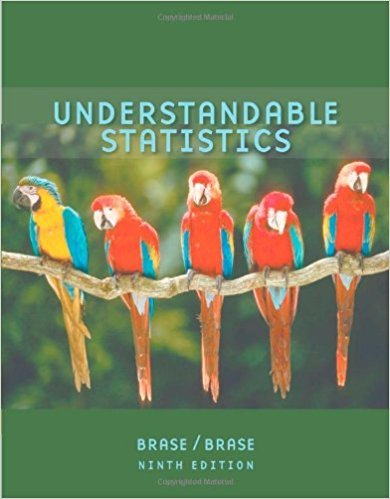×
×

# Solutions for Chapter 3.3: Organizing Data## Full solutions for Understandable Statistics | 9th Edition

ISBN: 9780618949922Solutions for Chapter 3.3: Organizing Data

Solutions for Chapter 3.3
4 5 0 411 Reviews
28
5
##### ISBN: 9780618949922

Chapter 3.3: Organizing Data includes 10 full step-by-step solutions. Understandable Statistics was written by and is associated to the ISBN: 9780618949922. Since 10 problems in chapter 3.3: Organizing Data have been answered, more than 35034 students have viewed full step-by-step solutions from this chapter. This textbook survival guide was created for the textbook: Understandable Statistics, edition: 9. This expansive textbook survival guide covers the following chapters and their solutions.

Key Statistics Terms and definitions covered in this textbook
• `-error (or `-risk)

In hypothesis testing, an error incurred by rejecting a null hypothesis when it is actually true (also called a type I error).

• Assignable cause

The portion of the variability in a set of observations that can be traced to speciic causes, such as operators, materials, or equipment. Also called a special cause.

• Attribute

A qualitative characteristic of an item or unit, usually arising in quality control. For example, classifying production units as defective or nondefective results in attributes data.

• Backward elimination

A method of variable selection in regression that begins with all of the candidate regressor variables in the model and eliminates the insigniicant regressors one at a time until only signiicant regressors remain

• Coeficient of determination

See R 2 .

• Conditional mean

The mean of the conditional probability distribution of a random variable.

• Conidence level

Another term for the conidence coeficient.

• Continuity correction.

A correction factor used to improve the approximation to binomial probabilities from a normal distribution.

• Deming

W. Edwards Deming (1900–1993) was a leader in the use of statistical quality control.

• Design matrix

A matrix that provides the tests that are to be conducted in an experiment.

• Enumerative study

A study in which a sample from a population is used to make inference to the population. See Analytic study

• Erlang random variable

A continuous random variable that is the sum of a ixed number of independent, exponential random variables.

• Error of estimation

The difference between an estimated value and the true value.

• Error sum of squares

In analysis of variance, this is the portion of total variability that is due to the random component in the data. It is usually based on replication of observations at certain treatment combinations in the experiment. It is sometimes called the residual sum of squares, although this is really a better term to use only when the sum of squares is based on the remnants of a model-itting process and not on replication.

• Error variance

The variance of an error term or component in a model.

• Expected value

The expected value of a random variable X is its long-term average or mean value. In the continuous case, the expected value of X is E X xf x dx ( ) = ?? ( ) ? ? where f ( ) x is the density function of the random variable X.

• Extra sum of squares method

A method used in regression analysis to conduct a hypothesis test for the additional contribution of one or more variables to a model.

• F distribution.

The distribution of the random variable deined as the ratio of two independent chi-square random variables, each divided by its number of degrees of freedom.

• Gamma function

A function used in the probability density function of a gamma random variable that can be considered to extend factorials

• Gamma random variable

A random variable that generalizes an Erlang random variable to noninteger values of the parameter r

×